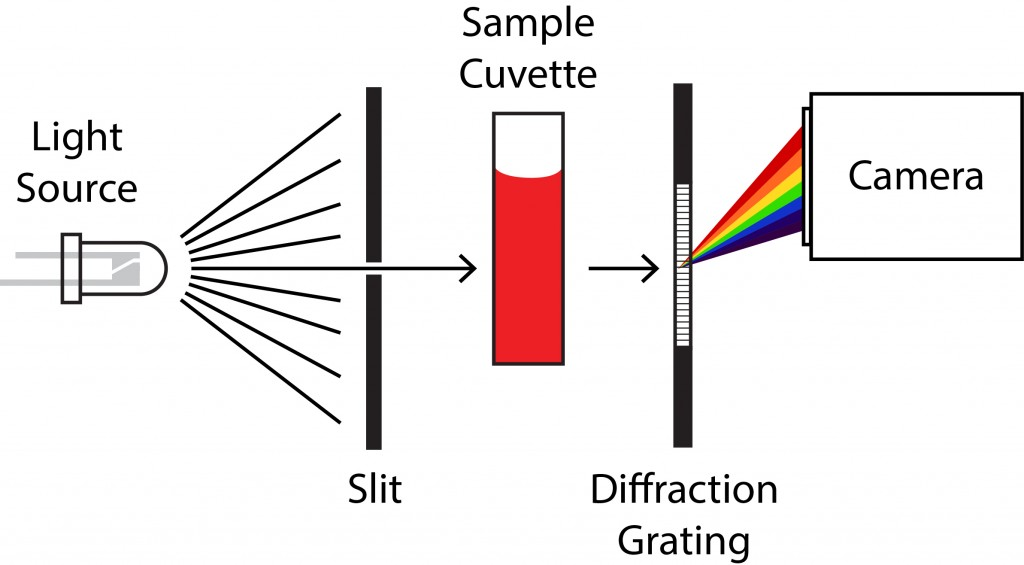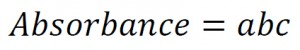# Spectroscopy Background

### What is visible absorption spectroscopy?

Absorption spectroscopy measures the amount of light absorbed by a sample at different wavelengths (λ). To quantify absorption, we compare the intensity of light passing through the spectrometer in the presence and absence of the sample. In other words, absorption is a relative value. As seen in the simplified diagram below, a white light source is sent through the sample and dispersed onto a detection camera. The light passing through the device without the sample has a measured intensity, I0, which is dependent on wavelength, I0(λ). With the sample in place, some of the light will be absorbed and the intensity of light at each wavelength is called I(λ).###### An absorbance spectrophotometer has a relatively simple light path. A light source is directed to the sample using a slit to isolate a well-defined beam of light. Light that passes through the sample cuvette is dispersed into its component colors by a transmission diffraction grating. The resulting spectrum is then recorded by a camera where the pixel position in the camera is related to wavelength.

To calculate absorbance we simply take the logarithm of the ratio of these intensities:The Beer-Lambert Law tells us that absorbance is linearly related to the concentration of the sample, c, the light path of the sample, b, and a proportionality constant called the absorptivity, a.* The Beer-Lambert Law simply states thatThe consequence of this relationship is that if one knows the absorptivity of a particular compound and the path length of the sample chamber, one can measure concentration with high precision. If the absorptivity is unknown, one can prepare a series of known concentrations of the compound to determine the it with high precision.

*Different fields will use different symbols, but abc is easy to remember. Common symbols in chemistry are ε,l,and c to refer to the absorptivity, path length and concentration respectively.